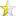Jan 14, 2008

# Making Games in C++In order to make a successful and user- friendly game in C++, we need to remember the following things:
First of all, simplicity is the key. Of course, if you are comfortable with the more advanced graphics capabilities of C++, you can go on to make a complex game such as Liero, but for now, the simpler the better.
Also, we need to remember that a game has to be the right difficulty- not too easy, not too hard. It needs also to have some sort of reward (e.g a colorful message) when you win, so the user is playing for some reason.
A game also needs to have a little more than plain text. For example, you could use a noughts and crosses board, or simply colorful text.

When you are comfortable with these concepts, you can go on to actually making the game.

If you are not familiar with outputting colored text, I suggest you learn how to do this before trying to make a game. It is actually very easy. First of all, just before you start the main process (before the `int main () {`), you need to add these lines:
 ``12345`` ``````void setcolor(unsigned short color) //The function that you'll use to { //set the colour HANDLE hcon = GetStdHandle(STD_OUTPUT_HANDLE); SetConsoleTextAttribute(hcon,color); }``````

then, before the command to output the text, you set the color with the command:
`setcolor (x);` (replace the (x) with any number e.g. setcolor (7) will come out with the default color, white.

Now, on to making the game. First of all, you need to have an idea of what kind of game you want to make. For the purpose of the tutorial, let us make a very simple game, called "Higher or Lower?". The user will be shown a number and asked if the next will be higher or lower.

First of all, we need to declare our variables. We should have three unsigned short integers. These should be the first number, the second number, and the overall score. We should then also have a character, which will be the letter the user enters, "H" or "L", higher or lower. We can declare these like this:
 ``123456`` ``````int main() { short unsigned int score = 0; short unsigned int num = 0; short unsigned int num2 = 0; char letter;``````

Now, in order to fully randomize the numbers which are outputted, we need to add in a few lines of code. These are as follows, with comments to explain which each line does.

 ``1234`` ``````loop: //This labels the code for quick reference later on. srand(time(NULL)); //Initialize random number generator num = 1 + rand() % (6 - 1 + 1); //Shows that num is a random integer between 1 and 6. num2 = 1 + rand() % (6 - 1 + 1); //Shows that num2 is a random integer between 1 and 6. ``````

 ``123456789`` ``````cout <<"\nPoints: "; setcolor (10); cout << score << endl; setcolor (7); cout <<"Get to 5 points to win. The numbers range between 1 and 6.\n"; setcolor (12); cout << num; setcolor (7); cout <<" is the first number. \nIs the next number going to be higher or lower? H or L?" << endl;``````
 ``123456789101112131415161718192021222324252627282930313233343536373839404142434445464748495051`` ``````cin >> letter; if (letter == 'h'||letter == 'H') { setcolor (12); cout << num2; setcolor (7); cout <<" is the second number."; if (num2>num) goto win; else if (num2num) goto lose; else if (num2==num) goto same; win: { if (score==4) { setcolor (12); cout <<" You completed the game! Well done!!!\n"; system ("pause"); return 0; } else {cout <<"You win! Well done!\n"; system ("pause"); score++; goto loop;} } same: {if (score==4) { setcolor (10); cout <<" You completed the game! Well done!!!\n"; system ("pause"); return 0;} else {cout <<"The numbers were the same! What a coincidence! I think\n we can give you a point for that..."; system ("pause"); score++; goto loop;}} lose: {cout <<"You lose...\n"; system ("pause"); }} return 0;}``````
 ``123456789101112131415161718192021222324252627282930313233343536373839404142434445464748495051525354555657585960616263646566676869707172737475767778798081`` ``````#include #include #include #include using namespace std; void setcolor(unsigned short color) //The function that you'll use to { //set the colour HANDLE hcon = GetStdHandle(STD_OUTPUT_HANDLE); SetConsoleTextAttribute(hcon,color); } int main() { short unsigned int score = 0; short unsigned int num = 0; short unsigned int num2 = 0; char letter; loop: srand(time(NULL)); //Initialize random number generator num = 1 + rand() % (6 - 1 + 1); num2 = 1 + rand() % (6 - 1 + 1); cout <<"\nPoints: "; setcolor (10); cout << score << endl; setcolor (7); cout <<"Get to 5 points to win. The numbers range between 1 and 6.\n"; setcolor (12); cout << num; setcolor (7); cout <<" is the first number. \nIs the next number going to be higher or lower? H or L?" << endl; cin >> letter; if (letter == 'h'||letter == 'H') { setcolor (12); cout << num2; setcolor (7); cout <<" is the second number."; if (num2>num) goto win; else if (num2num) goto lose; else if (num2==num) goto same; win: { if (score==4) { setcolor (12); cout <<" You completed the game! Well done!!!\n"; system ("pause"); return 0; } else {cout <<"You win! Well done!\n"; system ("pause"); score++; goto loop;} } same: {if (score==4) { setcolor (10); cout <<" You completed the game! Well done!!!\n"; system ("pause"); return 0;} else {cout <<"The numbers were the same! What a coincidence! I think\n we can give you a point for that..."; system ("pause"); score++; goto loop;}} lose: {cout <<"You lose...\n"; system ("pause"); }} return 0;}``````